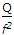# Materials and Components – Section 4

31. Lines of magnetic field cannot intersect.
 A. True B. False

32.  Assertion (A): Dielectric losses are proportional to tan δ.

Reason (R): tan δ =where ∈r and ∈r denote the real and imaginary parts of ∈r.
 A. Both A and R are true and R is correct explanation of A B. Both A and R are true but R is not correct explanation of A C. A is true but R is false D. A is false but R is true

33.  At T = 0, the resistivity of a perfect single crystal of a metal is
 A. 0 B. low C. high D. finite

Explanation:

At absolute zero temperature resistivity of metals is zero.

34.  In a polyatomic gas, the types of polarization which exist together are
 A. electronic and orientational B. electronic and ionic C. ionic and orientational D. electronic, orientational and ionic

Explanation:

All the three types of polarization exist in polyatomic gases.

35. Assertion (A): Metals are better heat conductors than insulators.

Reason (R): Ifis temperature gradient, Q is heat flow density and K is thermal conductivity, then Q = – K (dT/dx).

 A. Both A and R are true and R is correct explanation of A B. Both A and R are true but R is not correct explanation of A C. A is true but R is false D. A is false but R is true

36.  The number of tightly bound electrons in silicon atom are
 A. 14 B. 10 C. 6 D. 4

Explanation:

Silicon has 14 electons and 4 valence electrons. The 10 electrons in the inner orbits are tightly bound.

37.  Electric dipole moment is usually expressed in debye units. Here, 1 debye unit equals
 A. 3.33 x 10-30 C-m B. 3.33 x 10-30 C-m2 C. 3.30 x 10-10 C-m D. 3.33 x 10-10 C-m2

38.  A particle of charge Q coulombs is bound elastically to an equilibrium position with a force constant of f newton per metre. The polarizability of the system is
 A.B.C.D. Qf

39.  1 Bohr magneton =.
 A. True B. False